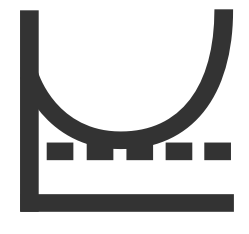Calculus

# Convergence - Problem Solving

If a sequence $\begin{array}{c}&& a_n = \frac{ p n^2 + q n + 2}{3n + 2 } \end{array}$ converges to $2,$ what is the value of $p +q?$

A sequence $a_n = \frac{3 n^p + 3 n^{p-1} }{3 n^{q} - 2 n^{q-1}}$ converges to 0 as $n\rightarrow\infty.$ Let the sequence $b_n$ be $\frac{1}{a_n}.$ Then which of the following is true about $b_n$ as $n\rightarrow\infty?$

Which of the following sequences does not converge as $n\rightarrow\infty:$ $\begin{array}{c}&a_n=\sin{2 n}, &a_n=\sin{3 \pi n}, &a_n=\frac{\sin{5n}}{n} , &a_n=\cos{ 4 \pi n}?\end{array}$

If a partial sum of the sequence $a_n$ $\sum _{ n=k }^{ k+5 }{ a_n }$ converges as $k\rightarrow\infty,$ what can we say about the sequence $a_n?$

If sequences $a_n$ and $b_n$ converge to $4$ and $3 ,$ respectively, which of the following is true about the sequence: $(4 a_n -1 ) ( 6 b_n +2 ) ?$

×